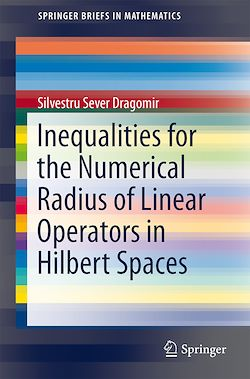# Inequalities for the Numerical Radius of Linear Operators in Hilbert Spaces

-English
120 Pages

Description

Aimed toward researchers, postgraduate students, and scientists in linear operator theory and mathematical inequalities, this self-contained monograph focuses on numerical radius inequalities for bounded linear operators on complex Hilbert spaces for the case of one and two operators. Students at the graduate level will learn some essentials that may be useful for reference in courses in functional analysis, operator theory, differential equations, and quantum computation, to name several. Chapter 1 presents fundamental facts about the numerical range and the numerical radius of bounded linear operators in Hilbert spaces. Chapter 2 illustrates recent results obtained concerning numerical radius and norm inequalities for one operator on a complex Hilbert space, as well as some special vector inequalities in inner product spaces due to Buzano, Goldstein, Ryff and Clarke as well as some reverse Schwarz inequalities and Grüss type inequalities obtained by the author. Chapter 3 presents  recent results regarding the norms and the numerical radii of two bounded linear operators. The techniques shown in this chapter are elementary but elegant and may be accessible to undergraduate students with a working knowledge of operator theory. A number of vector inequalities in inner product spaces as well as inequalities for means of nonnegative real numbers are also employed in this chapter. All the results presented are completely proved and the original references are mentioned.

Subjects

##### Differential equation

Informations